# ISEE Lower Level Quantitative : Quadrilaterals

## Example Questions

### Example Question #71 : Geometry

What is the perimeter of the polygon below?Possible Answers: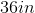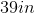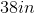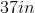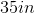Correct answer:Explanation:

To find the area of a perimeter, we add all of the side lengths together.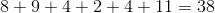### Example Question #22 : How To Find The Perimeter Of A Figure

What is the perimeter of the polygon below?Possible Answers: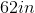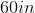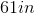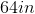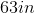Correct answer:Explanation:

To find the area of a perimeter, we add all of the side lengths together.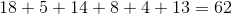### Example Question #23 : How To Find The Perimeter Of A Figure

What is the perimeter of the polygon below?Possible Answers: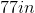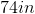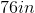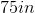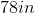Correct answer:Explanation:

To find the area of a perimeter, we add all of the side lengths together.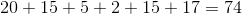### Example Question #72 : Geometry

What is the perimeter of the polygon below?Possible Answers: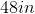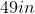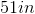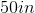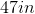Correct answer:Explanation:

To find the area of a perimeter, we add all of the side lengths together.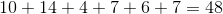### Example Question #32 : How To Find The Perimeter Of A Figure

What is the perimeter of the polygon below?Possible Answers: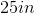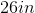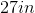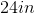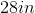Correct answer:Explanation:

To find the area of a perimeter, we add all of the side lengths together.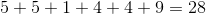### Example Question #33 : How To Find The Perimeter Of A Figure

What is the perimeter of the polygon below?Possible Answers: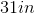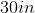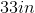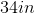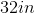Correct answer:Explanation:

To find the area of a perimeter, we add all of the side lengths together.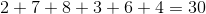### Example Question #34 : How To Find The Perimeter Of A Figure

What is the perimeter of the polygon below?Possible Answers: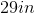Correct answer:Explanation:

To find the area of a perimeter, we add all of the side lengths together.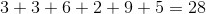### Example Question #35 : How To Find The Perimeter Of A Figure

What is the perimeter of the polygon below?Possible Answers: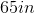Correct answer:Explanation:

To find the area of a perimeter, we add all of the side lengths together.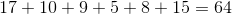### Example Question #36 : How To Find The Perimeter Of A Figure

What is the perimeter of the polygon below?Possible Answers:Correct answer:Explanation:

To find the area of a perimeter, we add all of the side lengths together.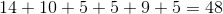### Example Question #37 : How To Find The Perimeter Of A Figure

What is the perimeter of the polygon below?Possible Answers:Correct answer:Explanation:

To find the area of a perimeter, we add all of the side lengths together.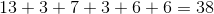### All ISEE Lower Level Quantitative Resources